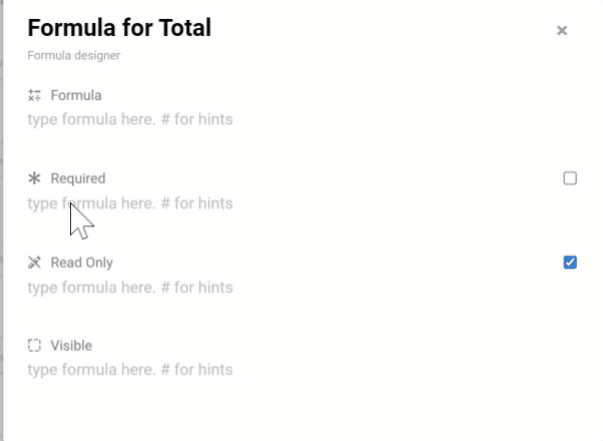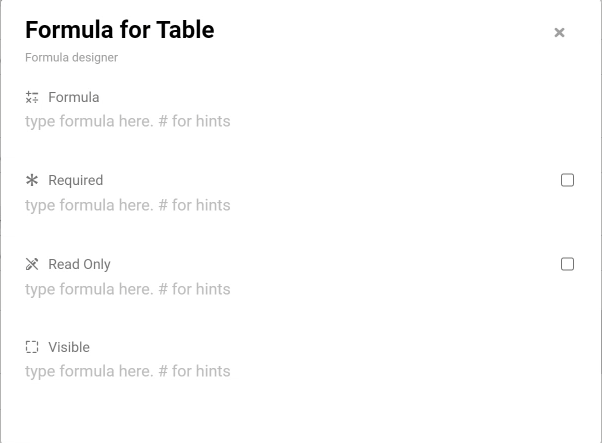# Formula Script Total/Sum - Updated

Here you can find how to use the new formula script

For example: Total = Number 1 + Number 2Result

For example: Amount = Unit Price x QuantityResult

For example: Total Amount = sum Amount from Table

Result